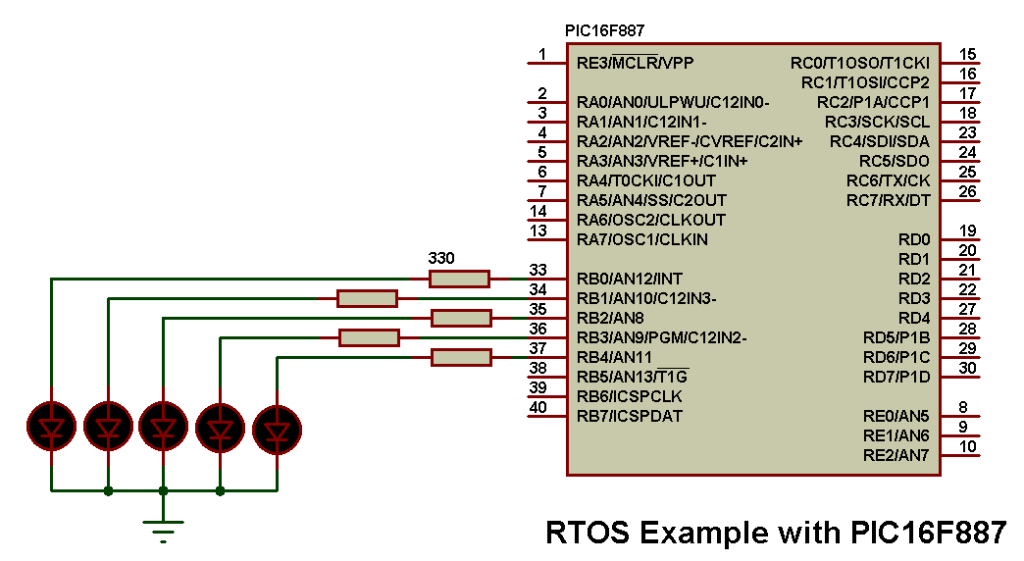# RTOS Example with PIC16F887 microcontroller and CCS C compiler

The RTOS (Real Time operating System) allows more than one task to run simultaneously (in parallel), for example reading from an analog channel, blinking an LED, setting the duty cycle of a PWM signal…..
This small article shows an RTOS example using PIC16F887 microcontroller and CCS PIC C compiler.

In this example we’ve 5 LEDs connected to the PIC16F887 microcontroller as shown in the circuit below. It is designed that the 5 LEDs are blinking but each one has its blinking frequency.In this example the PIC16F887 MCU uses its internal oscillator which is configured in the software @ 4MHz and MCLR pin function is disabled.

RTOS Example with PIC16F887 microcontroller CCS C code:
As mentioned above our RTOS has 5 LEDs with different frequencies (periods = 1/frequency).
The first LED which is connected to RB0 pin has the following task with a period of 500ms (250ms x 2):
#task(rate = 250ms, max = 50ms)
void led1(){
output_toggle(PIN_B0);
}
The same thing for the other LEDs.
In CCS C compiler the RTOS is configured using the following line:
#use rtos(timer = 0, minor_cycle = 50ms)
Here Timer0 is selected for the RTOS but other timers (Timer1 or Timer2) can also be used.
The complete C code is the one below.

The following video shows Proteus simulation of the RTOS example: